Question

5. How many milliliters of a 20% magnesium sulfate solution will contain 40 grams of the...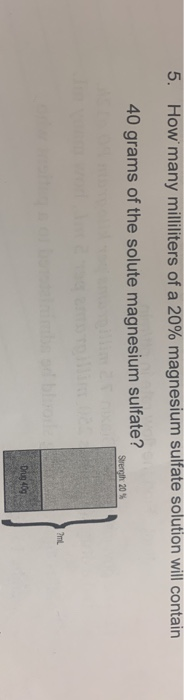5. How many milliliters of a 20% magnesium sulfate solution will contain 40 grams of the solute magnesium sulfate? Strength 20% Drug 409

1 ml of 20 % magnisium sulphate solution contain 200 mg

200 mg = 1 ml

4 gm=1ml/200mg

40,000mg=1/200*40,000

=200 ml

200 ml of 20% MgSO4 will contain 40 gm magnesium sulfate.

Which is Treatment of magnesium deficiency in hypomagnesaemia.

40 g or 160 mmols of magnesium ions (200ml of a 20% solution) by slow intravenous infusion (in glucose 5%) over up to 5 days, may be required to replace the deficit

Earn Coins

Coins can be redeemed for fabulous gifts.

Similar Homework Help Questions
• 3. How many milliliters of a 20% magnesium sulfate solution will contain 40 grams of the...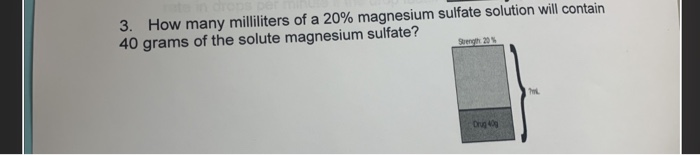3. How many milliliters of a 20% magnesium sulfate solution will contain 40 grams of the solute magnesium sulfate?

• 5.) How many milliliters of a 1.50 molar copper (II) sulfate solution contain 25.0 grams of...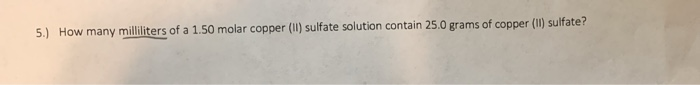5.) How many milliliters of a 1.50 molar copper (II) sulfate solution contain 25.0 grams of copper (II) sulfate?

• how many milliliters of 20% HBr solution contain 4 grams of HBr? 2. How many milliliters...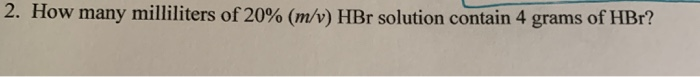how many milliliters of 20% HBr solution contain 4 grams of HBr? 2. How many milliliters of 20% (m/v) HBr solution contain 4 grams of HBr?

• 5. How many milliliters (mL) of a 3.277 M calcium nitrate solution would contain 76.50 grams...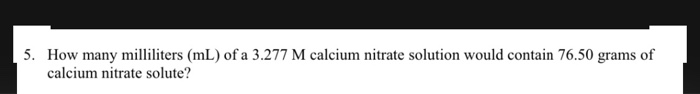5. How many milliliters (mL) of a 3.277 M calcium nitrate solution would contain 76.50 grams of calcium nitrate solute?

• A solution was found to contain 5.21% copper (II) sulfate. How many grams of solution would...

A solution was found to contain 5.21% copper (II) sulfate. How many grams of solution would contain 25.0 g of copper (II) sulfate?

• How many milliliters of a 12.5% dextrose solution contain 25 grams of dextrose? O A. 1,000...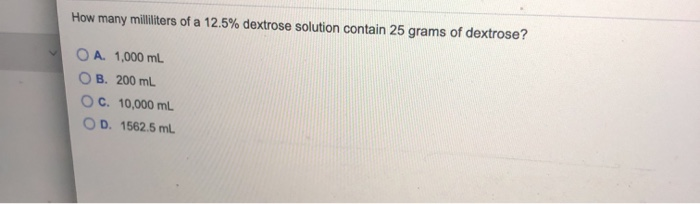How many milliliters of a 12.5% dextrose solution contain 25 grams of dextrose? O A. 1,000 mL O B. 200 ml O c. 10,000 ml OD. 1562.5 ml

• How many grams of magnesium sulfate should be added to 425 mL of de-ionized water to...

How many grams of magnesium sulfate should be added to 425 mL of de-ionized water to make a 0.075 M solution?

• How many milliliters of an aqueous solution of 0.182 M copper(II) sulfate is needed to obtain...

How many milliliters of an aqueous solution of 0.182 M copper(II) sulfate is needed to obtain 10.3 grams of the salt?

• How many grams of magnesium sulfate are needed to make 250.0 mL of a 0.250 M...

How many grams of magnesium sulfate are needed to make 250.0 mL of a 0.250 M solution? my response was a wrong answer 7.5 grams 0.250M *(250mL* 1L/1000mL)= 0.0625 0.0625* 120.4 g/mol (MgSO4)= 7.525 7.5 grams

• 2: How many milliliters of an aqueous solution of 0.219 M chromium(III) sulfate is needed to...

2: How many milliliters of an aqueous solution of 0.219 M chromium(III) sulfate is needed to obtain 3.70 grams of the salt? ____ml